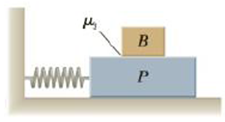Chapter 13, Problem 69AP

Chapter
Section
Textbook Problem

A large block P executes horizontal simple harmonic motion as it slides across a frictionless surface with a frequency f = 1.50 Hz. Block B rests on it, as shown in Figure P13.69, and the coefficient of static friction between the two is μs = 0.600. What maximum amplitude of oscillation can the system have if block B is not to slip?Figure P13.69

To determine
The maximum amplitude of oscillation the system can have for the block not to slip.

Explanation

Given info: The frequency of the harmonic motion is f=1.5Hz . The coefficient of static friction between the two blocks is μs=0.600 .

The maximum acceleration of simple harmonic motion is given by,

amax=Aω2 (1)

• amax is the maximum acceleration
• A is the maximum displacement
• ω is the angular frequency

The angular frequency is given by,

ω=2πf (2)

• f is the frequency of the oscillation

From (1) and (2),

amax=A(2πf)2 (3)

The maximum force that the block can sustain before slipping is given by,

fmax=μsn (4)

• μs is the coefficient of static friction
• n is the normal force on the block

The normal force on the block

n=mg (5)

• m is the mass of the object
• g is the free fall acceleration

From (4) and (5),

fmax=μsmg (6)

From Newton’s laws of motion, force is mass times acceleration

Still sussing out bartleby?

Check out a sample textbook solution.

See a sample solution

The Solution to Your Study Problems

Bartleby provides explanations to thousands of textbook problems written by our experts, many with advanced degrees!

Get Started

A deficiency of niacin may result in which disease? pellagra beriberi scurvy rickets

Nutrition: Concepts and Controversies - Standalone book (MindTap Course List)

25-32 What is the major groove of a DNA helix?

Introduction to General, Organic and Biochemistry

What impact has recombinant DNA technology had on genetics and society?

Human Heredity: Principles and Issues (MindTap Course List)